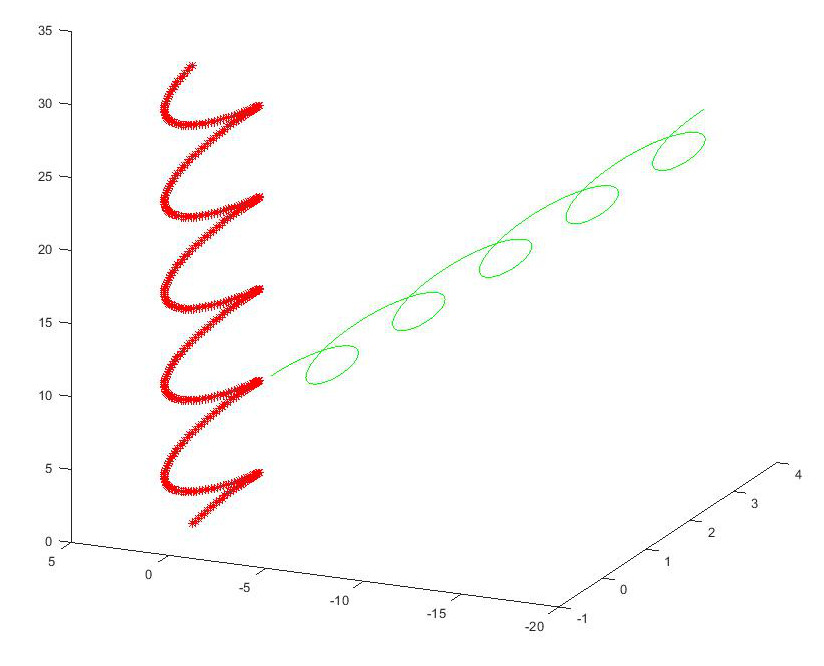# Tutorial using Matlab to show how to perform transforms in 3D from one coordinate system to another

For real world vision or Robotic operations it is sometimes necessary to transform between coordinates in different coordinate systems. In this tutorial we will transform a 3D spiral from one coordinate system to another. The coordinate systems are specified in terms of their position in Cartesian space and converted to a vectorial representation.

First let us create and display the spiral

``````
t = 0:pi/50:10*pi;
st = sin(t);
ct = cos(t);
``````

The spiral is in 3D but we need a 4D matrix to perform the matrix transformations, so we also add a row of ones to it

``````
spiral = [st; ct; t; ones(501,1)']
``````

Ok, lets plot the relevant data

``````
plot3(spiral(1,:), spiral(2,:), spiral(3,:), 'b')
``````

we use the hold on command to keep plotting to the same chart

``````
hold on
``````

We are going to translate this spiral from its world coordinates to another local coordinate system

the coordinates are specified by a matrix = [X1, X2, X3, Y1, Y2, Y3, Z1, Z2, Z3]

``````
world = [1,0,0;0,1,0;0,0,1]
local = [3,6,3;3,3,6;3,3,0]
``````

The 3,3,0 in the last row (Z axis) of the matrix creates a 45 degree plane

Lets turn our local coordinate system into a vector

``````
local_vector = zeros(3,3)
local_vector(:,1) = local(:,2)-local(:,1)
local_vector(:,2) = local(:,3)-local(:,1)
local_vector(:,3) = cross(local_vector(:,1),local_vector(:,2))
``````

The vectors should be normalised to unit length like our world vector is

``````
local_vector(:,1) = local_vector(:,1)/norm(local_vector(:,1))
local_vector(:,2) = local_vector(:,2)/norm(local_vector(:,2))
local_vector(:,3) = local_vector(:,3)/norm(local_vector(:,3))
``````

Now we have to create our transform matrix using the dot product between our world and local vectors, this sets up the skew, reflection, rotation elements

``````
transform = zeros(4,4)
transform(1,1) = dot(world(1,:), local_vector(1,:))
transform(1,2) = dot(world(1,:), local_vector(2,:))
transform(1,3) = dot(world(1,:), local_vector(3,:))
transform(2,1) = dot(world(2,:), local_vector(1,:))
transform(2,2) = dot(world(2,:), local_vector(2,:))
transform(2,3) = dot(world(2,:), local_vector(3,:))
transform(3,1) = dot(world(3,:), local_vector(1,:))
transform(3,2) = dot(world(3,:), local_vector(2,:))
transform(3,3) = dot(world(3,:), local_vector(3,:))
``````

we also add the translated origin of our coordinate system to the last column of the matrix, this performs the X,Y,Z translation

``````
transform(1,4) = local(1,1)
transform(2,4) = local(2,1)
transform(3,4) = local(3,1)
transform(4,4) = 1
``````

So using our transform we can rotate the spiral by 45 degrees and translate it by 3 in X and 3 in Y

``````
tspiral = transform*spiral;
``````

let’s plot it in green next to the original spiral

``````
plot3(tspiral(1,:), tspiral(2,:), tspiral(3,:), 'g')
``````

(you might want to rotate the plot axis now to see the two spirals in 3D)

now to get it back again we use the inverse of our transformation matrix

``````
tunspiralInv = inv(transform)*tspiral;
``````

and plot it using markers so we can see the orginal spiral beneath

plot3(tunspiralInv(1,:), tunspiralInv(2,:), tunspiralInv(3,:), ‘r*’)

The plot should look like thisFor the mathematical details behind the transform, these MIT lecture notes provide an excellent explanation.

If you find the article useful, please feel free to post a link to it